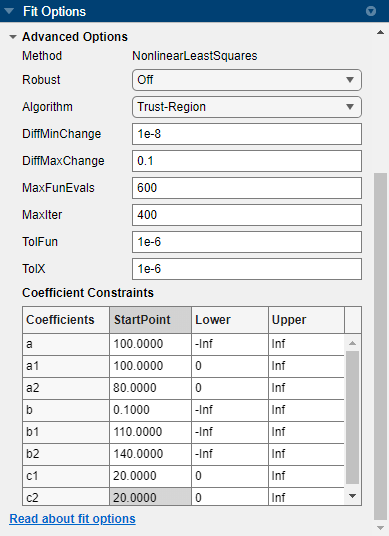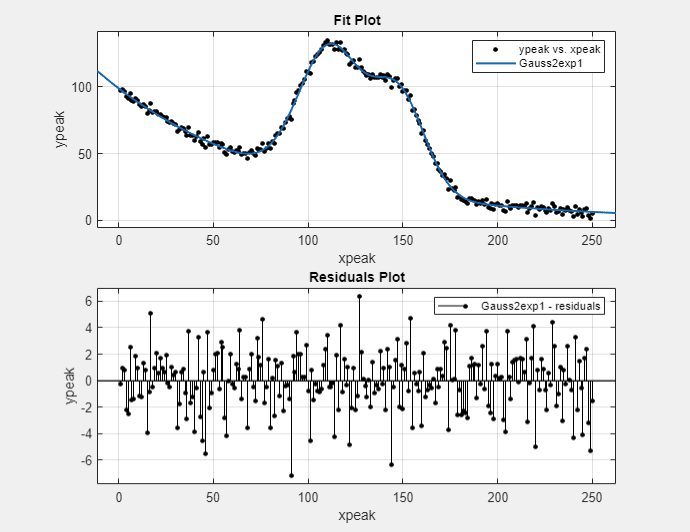## Gaussian Fitting with an Exponential Background

This example fits two poorly resolved Gaussian peaks on a decaying exponential background using a general (nonlinear) custom model.

Fit the data using this equation

`$y\left(x\right)=a{e}^{-bx}+{a}_{1}{e}^{-{\left(\frac{x-{b}_{1}}{{c}_{1}}\right)}^{2}}+{a}_{2}{e}^{-{\left(\frac{x-{b}_{2}}{{c}_{2}}\right)}^{2}}$`

where ai are the peak amplitudes, bi are the peak centroids, and ci are related to the peak widths. Because unknown coefficients are part of the exponential function arguments, the equation is nonlinear.

1. Load the data and open the Curve Fitter app.

```load gauss3 curveFitter```

The workspace contains two new variables:

• `xpeak` is a vector of predictor values.

• `ypeak` is a vector of response values.

2. In the Curve Fitter app, on the Curve Fitter tab, in the Data section, click . In the Select Fitting Data dialog box, select `xpeak` as the X Data value and `ypeak` as the Y Data value. Enter `Gauss2exp1` as the Fit name value.

3. On the Curve Fitter tab, in the Fit Type section, click the arrow to open the gallery. In the fit gallery, click Custom Equation in the Custom group.

4. In the Fit Options pane, replace the example text in the equation edit box with these terms:

`a*exp(-b*x) + a1*exp(-((x-b1)/c1)^2) + a2*exp(-((x-b2)/c2)^2)`

The fit is poor (or incomplete) at this point because the starting points are randomly selected and no coefficients have bounds.

5. Specify reasonable coefficient starting points and constraints. Deducing the starting points is particularly easy for the current model because the Gaussian coefficients have a straightforward interpretation and the exponential background is well defined. Additionally, as the peak amplitudes and widths cannot be negative, constrain a1, a2, c1, and c2 to be greater than 0.

1. In the Fit Options pane, click Advanced Options.

2. In the Coefficient Constraints table, change the Lower bound for a1, a2, c1, and c2 to `0`, as the peak amplitudes and widths cannot be negative.

3. Enter the StartPoint values as shown for the specified coefficients.

CoefficientsStart Point
`a`100
`a1`100
`a2`80
`b`0.1
`b1`110
`b2`140
`c1`20
`c2`20As you change the fit options, the Curve Fitter app updates the fit.

6. Observe the fit and residuals plots. To create a residuals plot, click in the Visualization section of the Curve Fitter tab.